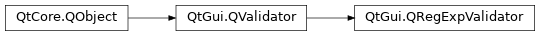# QRegExpValidator¶

The `QRegExpValidator` class is used to check a string against a regular expression. More## Detailed Description¶

`QRegExpValidator` uses a regular expression (regexp) to determine whether an input string is `Acceptable` , `Intermediate` , or `Invalid` . The regexp can either be supplied when the `QRegExpValidator` is constructed, or at a later time.

When `QRegExpValidator` determines whether a string is `Acceptable` or not, the regexp is treated as if it begins with the start of string assertion (^ ) and ends with the end of string assertion (\$ ); the match is against the entire input string, or from the given position if a start position greater than zero is given.

If a string is a prefix of an `Acceptable` string, it is considered `Intermediate` . For example, “” and “A” are `Intermediate` for the regexp [A-Z][0-9] (whereas “_” would be `Invalid` ).

For a brief introduction to Qt’s regexp engine, see `QRegExp` .

Example of use:

```# regexp: optional '-' followed by between 1 and 3 digits
rx = QRegExp("-?\\d{1,3}")
validator = QRegExpValidator(rx, self)

edit = QLineEdit(self)
edit.setValidator(validator)
```

Below we present some examples of validators. In practice they would normally be associated with a widget as in the example above.

```# integers 1 to 9999
rx = QRegExp("[1-9]\\d{0,3}")
# the validator treats the regexp as "^[1-9]\\d{0,3}\$"
v = QRegExpValidator(rx, 0)
pos = 0

s = "0"
v.validate(s, pos)    # returns Invalid
s = "12345"
v.validate(s, pos)    # returns Invalid
s = "1"
v.validate(s, pos)    # returns Acceptable

rx.setPattern("\\S+")            # one or more non-whitespace characters
v.setRegExp(rx)
s = "myfile.txt"
v.validate(s, pos) # Returns Acceptable
s = "my file.txt"
v.validate(s, pos) # Returns Invalid

# A, B or C followed by exactly five digits followed by W, X, Y or Z
rx.setPattern("[A-C]\\d{5}[W-Z]")
v.setRegExp(rx)
s = "a12345Z"
v.validate(s, pos)        # Returns Invalid
s = "A12345Z"
v.validate(s, pos)        # Returns Acceptable
s = "B12"
v.validate(s, pos)        # Returns Intermediate

rx.setCaseSensitive(false)
v.setRegExp(rx)
v.validate(s, pos) # Returns Acceptable
v.validate(s, pos) # Returns Acceptable
v.validate(s, pos) # Returns Invalid
v.validate(s, pos) # Returns Intermediate
```

`QRegExp` `QIntValidator` `QDoubleValidator` Settings Editor Example

class PySide2.QtGui.QRegExpValidator([parent=None])

PySide2.QtGui.QRegExpValidator(rx[, parent=None])

param parent:

`PySide2.QtCore.QObject`

param rx:

`PySide2.QtCore.QRegExp`

Constructs a validator with a `parent` object that accepts any string (including an empty one) as valid.

Constructs a validator with a `parent` object that accepts all strings that match the regular expression `rx` .

The match is made against the entire string; e.g. if the regexp is [A-Fa-f0-9]+ it will be treated as ^[A-Fa-f0-9]+\$ .

PySide2.QtGui.QRegExpValidator.regExp()
Return type:

`PySide2.QtCore.QRegExp`

This property holds the regular expression used for validation.

By default, this property contains a regular expression with the pattern `.*` that matches any string.

PySide2.QtGui.QRegExpValidator.regExpChanged(regExp)
Parameters:
PySide2.QtGui.QRegExpValidator.setRegExp(rx)
Parameters:

This property holds the regular expression used for validation.

By default, this property contains a regular expression with the pattern `.*` that matches any string.